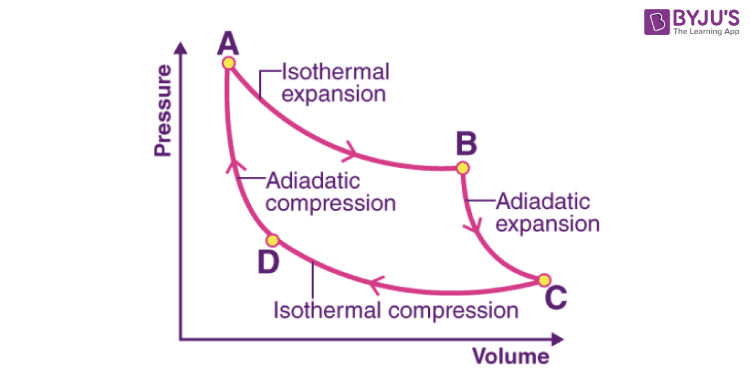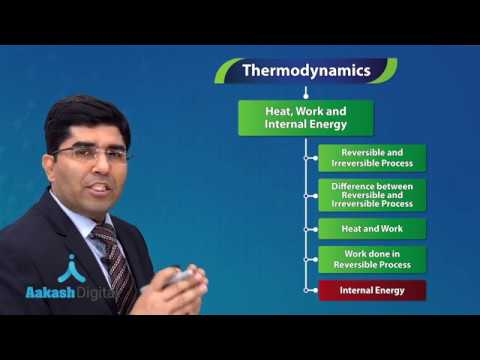Jet Set Go! All about Aeroplanes Jet Set Go! All about Aeroplanes

# Internal Energy Questions

A number of thermodynamic quantities appear during the study of thermodynamics. For example, internal energy, heat, work, enthalpy, entropy, free energy etc. The internal energy is well known whenever some process occurs; it is usually accompanied by some energy change. Energy may appear in different forms such as heat, light and work etc.

 Definition: The internal energy change is the heat evolved or absorbed at a constant volume.

## Internal Energy Chemistry Questions with Solutions

Q-1: The internal energy is the heat evolved or absorbed at _________.

a) Constant pressure

b) Constant volume

c) Constant temperature

d) Constant moles

Explanation: The heat that is emitted or absorbed at a constant volume is the internal energy.

Q-2: The internal energy always has a _________.

a) Indefinite value

b) Definite value

c) Both of the above

d) Can’t be predicted

Explanation: The internal energy of a definite amount of a particular substance under given conditions of temperature and pressure always has a definite value.

Q-3: Why can the exact or absolute value of internal energy not be determined?

Answer: It is impossible to determine an object’s internal energy in its exact or absolute form. This is because most of the quantities that contribute to the system’s internal energy are impossible to estimate with accuracy.

Q-4: Which of the following laws explained how internal energy, heat, and work relate to one another?

a) Zeroth law of thermodynamics

b) Second law of thermodynamics

c) Third law of thermodynamics

d) First law of thermodynamics

Explanation: According to the first law of thermodynamics, ΔE = q + w

Where q is either heat absorbed or given out by the system.

W is the work done on or by the system.

Q-5: Calculate the internal energy change in each of the following cases:

(i) A system absorbs 25 kJ of heat and does 15 kJ of work.

ii) 15 kJ of work is done on the system, and 25 kJ of heat is given out by the system.

(i) Here, q= +25 kJ and w= -15 kJ

According to the first law of thermodynamics,

ΔE = q + w = +25 -15 = 10 kJ

This implies that the internal energy of the system increases by 10 kJ.

ii) Here, q= -25 kJ and w= +15 kJ

According to the first law of thermodynamics,

ΔE = q + w = -25 +15 = -10 kJ

This implies that the internal energy of the system decreases by 10 kJ.

Q-6: Internal energy is _________.

a) Partly potential and partly kinetic

b) Totally kinetic

c) Totally potential

d) None of the above

Answer: a) Partly potential and partly kinetic

Q-7: Internal energy (ΔE) is equal to the heat supplied(q) in _________.

b) Isochoric change

c) Isothermal reversible change

d) Isothermal irreversible change

Explanation: For Isochoric change, ΔV=0

w= PΔV = 0

Since, ΔE = q + w

This implies, ΔE = q

Q-8: A chemist, while studying the properties of gaseous CCI2F2, a chlorofluorocarbon refrigerant, cooled a 1.25 g sample at constant atmospheric pressure of 1.0 atm from 320 K to 293 K. During cooling, the sample volume decreased from 274 to 248 mL. Calculate ΔE for the chlorofluorocarbon for this process. For CCI2F2, Cp= 80.7 J/(mol K).

Answer: ΔH = qp, and Cp is the heat evolved or absorbed per mole of 1o fall or rise in temperature.

Here, fall in temperature = 320-293 = 27 K.

Molar mass of CCI2F2 = 121 g/mol

Therefore, the heat evolved from 1.25 g of the sample on being cooled from 320 K to 293 K at constant pressure = (80.7/121) × 1.25 × 27 J = 22.51 J.

Further ΔH = ΔU + PΔV = -22.51 J

PΔV = 1 atm × [(248-274)/1000] L = -0.026 L atm = -0.026 × 101.35 J = -2.63 J

Therefore, -22.51 = ΔU + (-2.63)

ΔU = -19.88 J

Q-9: Calculate the standard internal energy change for the reaction OF2 (g) + H2O (g)→ O2 (g) + 2HF (g) at 298 K, given that the enthalpies of formation of OF2(g), H2O(g) and HF(g) are +20, -250 and -270 kJ/mol, respectively.

Step 1: Calculation of standard enthalpy of reaction.

ΔHfo= ∑ΔHfo(products) – ∑ΔHfo(reactants)

ΔHfo= [ΔHfo( O2) + 2ΔHfo(HF)] – [ΔHfo( OF2) + ΔHfo(H2O)]

ΔHfo= [0 + 2(-270)] – [+20 + (-250)] kJ/mol

ΔHfo= -310 kJ/mol

Step 2: Calculation of standard internal energy change.

For the given reaction,

Δng= (1+2) – (1+1) = 1

ΔHo = ΔUo + ΔngRT

Or ΔUo = ΔHo – ΔngRT

ΔUo = -310 – [(1) × (8.314× 10-3 kJK-1mol-1) × (298 K)]

ΔUo = -312.48 kJmol-1

Q-10: For which of the following reactions ΔH = ΔU?

a) N2(g) + 3H2(g) → 2NH3(g)

b) PCl3(g) + Cl2(g) → PCl5(g)

c) H2(g) + I2(g) → 2HI(g)

d) 2KClO3(s) → 2KCl(s) + 3O2(g)

Answer: c) H2(g) + I2(g) → 2HI(g)

Explanation: As we know, ΔH = ΔU + ΔngRT

Where Δng denotes the stoichiometric difference between the gaseous products and the gaseous reactants.

Chemical Reactions Δng
N2(g) + 3H2(g) → 2NH3(g 2-4 = -2
PCl3(g) + Cl2(g) → PCl5(g) 1-2 = -1
H2(g) + I2(g) → 2HI(g) 2-2 = 0
2KClO3(s) → 2KCl(s) + 3O2(g) 3-0 = 3

Hence for reaction c) ΔH = ΔU as Δng= 0.

Q-11: In an isochoric process, the increase in internal energy is _________.

a) Equal to the heat absorbed.

b) Equal to the heat evolved.

c) Equal to the work done.

d) Equal to the sum of the heat absorbed and work done.

Answer:a) Equal to the heat absorbed.

Explanation: For isochoric processes, ΔV = 0, so qV = ΔE, i.e. heat given to a system under constant volume is used up in increasing ΔE.

Q-12: How do ΔU and ΔW correspond for the reaction of one mole of HCl with zinc dust in a bomb calorimeter?

Answer: The bomb calorimeter, which consists of a sealed combustion chamber known as a bomb, is frequently used to measure the heat of combustion of organic substances. No expansion or compression is allowed during a procedure if the container is sealed. So W = 0 and ΔU = q.

Q-13: Which of the following is true for isothermal expansion?

a) ΔV = 0

b) ΔA = 0

c) ΔG = 0

d) ΔU = 0

Explanation: When the temperature remains constant, isothermal expansion occurs. This suggests that ΔT = 0. Additionally, since internal energy depends on temperature, isothermal expansion results in zero internal energy.

Q-14: For the reaction X2O4 (l) → 2XO2 (g) at 298 K, given the values, ΔU = 9 kJ and ΔS = 84 JK-1. Calculate ΔG.

Answer: Given reaction is X2O4 (l) → 2XO2 (g), T= 298 K

ΔU = 9 kJ and ΔS = 84 JK-1, ΔH = ?

Δng= 2-0 = 2

ΔH = ΔU+ ΔngRT = 9kJ + 2×8.314×298 = (9000 J + 4955.144) J = 13955.144 J

ΔG = ΔH – TΔS = 13955.144 J – (298 K × 84 JK-1) = -11076.856 J = -11.08 kJ

Q-15: From the below Carnot cycle undergone by an ideal gas, identify the processes in which the change in internal energy is non-zero.Answer: Internal energy (ΔU) is a function of temperature (ΔT) since the temperature remains constant during an isothermal process, so the change in internal energy is zero for an isothermal process.

For adiabatic processes (expansion as well as compression), q is zero, but ΔT is not. So, ΔU is also non-zero.

## Practice Questions on Internal Energy

Q-1: A system received a continuous supply of 600 joules of heat at constant volume. The system’s temperature rose from 30 to 35 degrees Celsius as a result. In what ways has the system’s internal energy changed?

Q-2: Which energy is not part of the internal energy?

a) Nuclear energy

b) Chemical bond energy

c) Gravitational energy

d) Potential energy

Q-3: ΔU is zero in

a) Isobaric process

b) Cyclic process

d) Isochoric process

Q-4: The molar enthalpy change for the condensation of 1 mol of water at 1 bar and 100 °C is 51kJ/mol if water vapour is taken to be a perfect gas. Determine the internal energy when 1 mol of water condenses at 100 °C and 1 bar of pressure.

Q-5: Why is it preferable to determine the change in enthalpy rather than the change in internal energy?

Click the PDF to check the answers for Practice Questions.

## Thermodynamics – Heat, Work and Internal Energy